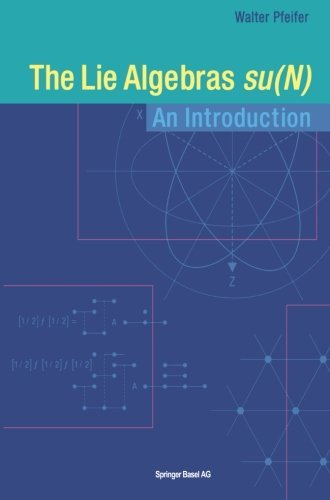### The Lie Algebras su(N), an Introduction

2003 (revised 2008), ISBN 3-7643-2418-XThe su(N) Lie algebras very frequently appear and "there is hardly any student of physics or mathematics who will never come across symbols like su(2) and su(3)" (Fuchs, Schweigert, 1997, p. XV). For instance, the algebra su(2) describes angular momenta, su(3) is related to harmonic oscillator properties or to rotation properties of systems and su(4) represents states of elementary particles in the quark model.

This book is mainly directed to undergraduate students of physics or to interested physicists. It is conceived to give directly a concrete idea of the su(N) algebras and of their laws. The detailed developments, the numerous references to preceding places, the figures and many explicit calculations of matrices should enable the beginner to follow. Laws which are given without proof are marked clearly and mostly checked with numerical tests. Knowledge of basic linear algebra is a prerequisite. Many results are obtained, which hold generally for (simple) Lie algebras. Therefore, the text on hand can make the lead-in to this field easier.

The structure of the contents is simple. First, Lie algebras are defined and the su(N) algebras are introduced starting from antihermitian matrices. In chapter 3, the su(2) algebras, their multiplets and the direct product of the multiplets are investigated. The treatment of the su(3) multiplets in chapter 4 is more labour-intensive. Casimir operators and methods to determine the dimensions of multiplets are introduced. In chapter 5, the su(4) multiplets are represented three-dimensionally. Making use of the su(4) algebra, the properties of the Cartan-Weyl basis are demonstrated. Chapter 6 points to general relations of the su(N) algebras.